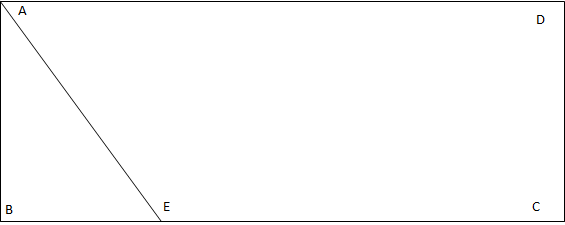Question 14

# In the figure given below, ABCD is a rectangle. The area of the isosceles right triangle ABE = 7 $$cm^2$$ ; EC = 3(BE). The area of ABCD (in $$cm^2$$) isSolutionLet AB = BE = x

Area of triangle ABE = $$x^2/2$$ = 14; we get x = $$\sqrt{14}$$

So we have side BC = 4*$$\sqrt{14}$$

Now area is AB*BC = 14 *4 = 56 $$cm^2$$